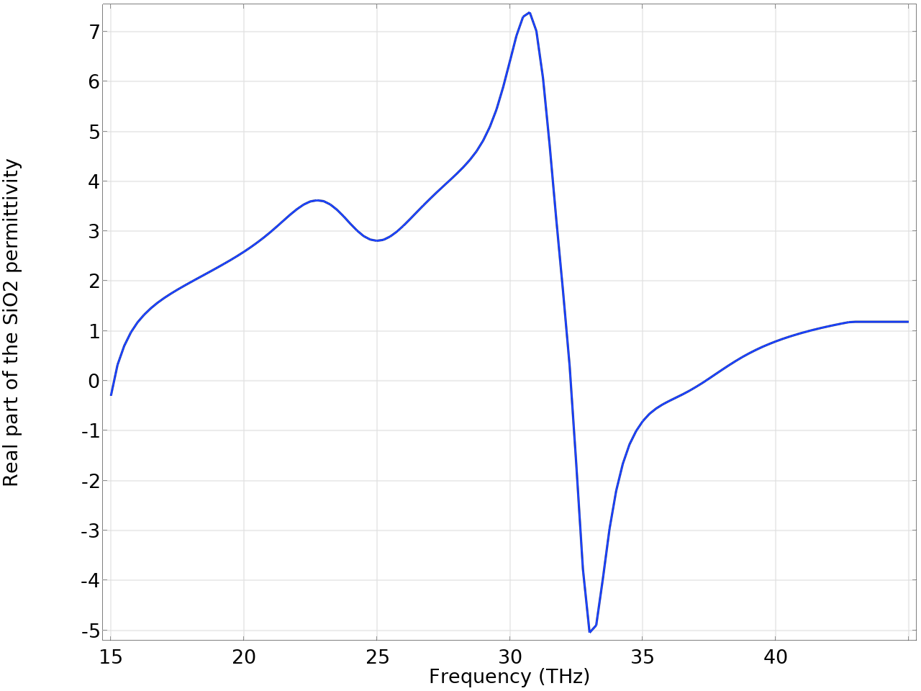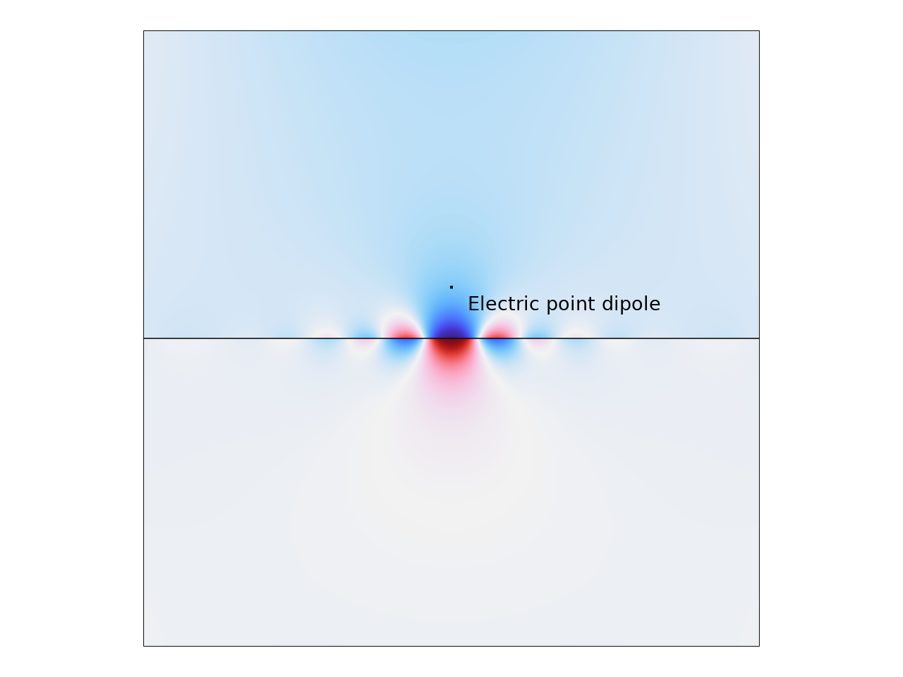# 在 COMSOL® 中对表面等离激元进行建模

2022年 10月 12日

### 最简单的等离激元色散的推导(1)

H^=(0,0,H_z^)e^{j(\omega t – k_{SPP}x)}e^{-k_y^+ y}

(2)

E^=(E_x^+,E_y^+,0)e^{j(\omega t – k_{SPP}x)}e^{-k_y^+ y}

(3)

H^=(0,0,H_z^)e^{j(\omega t – k_{SPP}x)}e^{k_y^- y}

(4)

E^=(E_x^-,E_y^-,0)e^{j(\omega t – k_{SPP}
x)}e^{k_y^- y}

(5)

-jk_{SPP}
E_x = k_y^+\frac{D_y}{\varepsilon_d}

(6)

-jk_{SPP}E_x=k_y^-\frac{D_y} {\varepsilon_m}.

(7)

\frac{k_y^+}{\varepsilon_d}=-\frac{k_y^-}{\varepsilon_m}
.

(8)

k_{SPP}^2=\varepsilon_d k_0^2-k_y^{+2}

(9)

k_{SPP}^2=\varepsilon_m k_0^2-k_y^{-2}

(10)

k_{SPP}= \sqrt{\frac{\varepsilon_d \varepsilon_m}{\varepsilon_d+\varepsilon_m}}k_0.

### 模拟表面等离激元的传播和色散### 金属薄膜中的表面等离激元### 新型 2D 材料中的表面等离激元SiO2 的实部红外频率的介电常数。由于声子共振，介电常数在 33 THz 左右变为负，其中石墨烯表面等离激元不受支持。### 参考文献

1. S. A. Maier, Plasmonics: fundamentals and applications. Springer, 2007.

#### 评论 (16)

##### 留言##### 江 樊
2022-11-20##### Min Yuan
2023-04-12 COMSOL 员工##### 子昂 徐
2023-04-04##### Min Yuan
2023-04-12 COMSOL 员工##### Joe B
2023-04-24##### Min Yuan
2023-06-27 COMSOL 员工##### 聊新 孙
2023-06-13

MoO3的案例 能给一下 让我们学习吗##### Min Yuan
2023-06-27 COMSOL 员工##### Xuan Liu
2023-06-15

Mark, 先马后学##### pw li
2023-06-26

MoO3的案例 能给一下 让我们学习吗##### Min Yuan
2023-06-27 COMSOL 员工##### ka ka
2023-09-09##### Min Yuan
2023-09-13 COMSOL 员工##### 央 未
2023-09-13##### Min Yuan
2023-09-20 COMSOL 员工2023-09-27